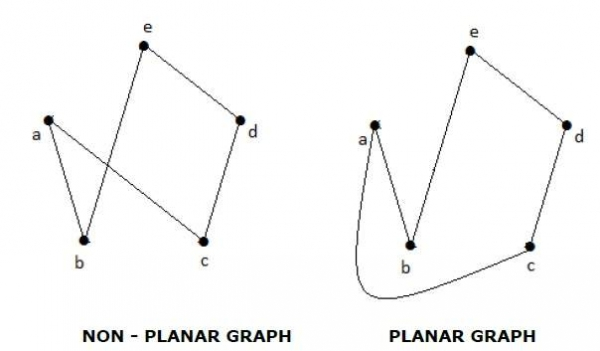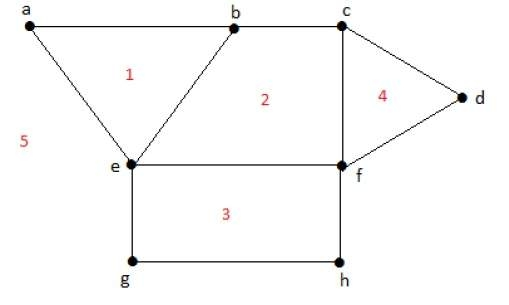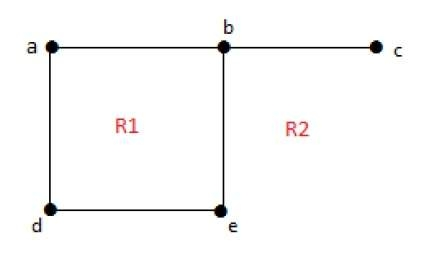# Planar Graphs and their Properties

A graph 'G' is said to be planar if it can be drawn on a plane or a sphere so that no two edges cross each other at a non-vertex point.

## Example## Regions

Every planar graph divides the plane into connected areas called regions.

## ExampleDegree of a bounded region r = deg(r) = Number of edges enclosing the regions r.

deg(1) = 3
deg(2) = 4
deg(3) = 4
deg(4) = 3
deg(5) = 8Degree of an unbounded region r = deg(r) = Number of edges enclosing the regions r.

deg(R1) = 4
deg(R2) = 6

In planar graphs, the following properties hold good −

• 1. In a planar graph with 'n' vertices, sum of degrees of all the vertices is

n ∑ i=1 deg(Vi) = 2|E|
• 2. According to Sum of Degrees of Regions Theorem, in a planar graph with 'n' regions, Sum of degrees of regions is −

n ∑ i=1 deg(ri) = 2|E|

Based on the above theorem, you can draw the following conclusions −

In a planar graph,

• If degree of each region is K, then the sum of degrees of regions is

K|R| = 2|E|

• If the degree of each region is at least K(≥ K), then

K|R| ≤ 2|E|

• If the degree of each region is at most K(≤ K), then

K|R| ≥ 2|E|

Note − Assume that all the regions have same degree.

3. According to Euler's Formulae on planar graphs,

• If a graph 'G' is a connected planar, then

|V| + |R| = |E| + 2

• If a planar graph with 'K' components then

|V| + |R|=|E| + (K+1)

Where, |V| is the number of vertices, |E| is the number of edges, and |R| is the number of regions.

4. Edge Vertex Inequality

If 'G' is a connected planar graph with degree of each region at least 'K' then,

|E| ≤ k/k - 2{|v|-2}

You know, |V| + |R| = |E| + 2

K.|R| ≤ 2|E|

K(|E| - |V| + 2) ≤ 2|E|

(K - 2)|E| ≤ K(|V| - 2)

|E| ≤ k/k - 2{|v| - 2}

5. If 'G' is a simple connected planar graph, then

|E| ≤ 3|V| − 6
|R| ≤ 2|V| − 4

There exists at least one vertex V ∈ G, such that deg(V) ≤ 5

6. If 'G' is a simple connected planar graph (with at least 2 edges) and no triangles, then

|E| ≤ {2|V| – 4}

7. Kuratowski's Theorem

A graph 'G' is non-planar if and only if 'G' has a subgraph which is homeomorphic to K5 or K3,3.